# Just Beatty It

[This is the 6th post in the current series about Wythoff’s game: see posts #1, #2, #3, #4, and #5. Caveat lector: this post is a bit more difficult than usual. Let me know what you think in the comments!]

Our only remaining task from last week was to prove the mysterious Covering Theorem: we must show that there is exactly one dot in each row and column of the grid (we already covered the diagonal case). Since the rows and columns are symmetric, let’s focus on columns.

The columns really only care about the x-coordinates of the points, so let’s draw just these x-coordinates on the number-line. We’ve drawn $$\phi,2\phi,3\phi,\ldots$$ with small dots and $$\phi^2,2\phi^2,3\phi^2,\ldots$$ with large dots. We need to show that there’s exactly one dot between 1 and 2, precisely one dot between 2 and 3, just one between 3 and 4, and so on down the line. For terminology’s sake, break the number line into length-1 intervals [1,2], [2,3], [3,4], etc., so we must show that each interval has one and only one dot: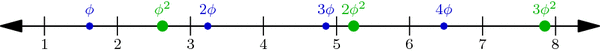Multiples of $$\phi$$ (small blue dots) and of $$\phi^2$$ (large green dots) perfectly interleave the integers on the number line. This is proved below.

Why is this true? One explanation hinges on a nice geometric observation: Take any small dot s and large dot t on our number-line above, and cut segment st into two parts in the ratio $$1:\phi$$ (with s on the shorter side). Then the point where we cut is always an integer! For example, the upper-left segment in the diagram below has endpoints at $$s=2\cdot\phi$$ and $$t=1\cdot\phi^2$$, and its cutting point is the integer 3: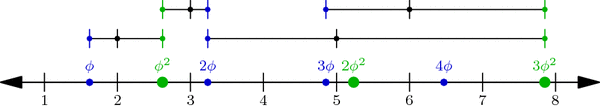When a segment formed by a small and a large dot is cut into a $$1:\phi$$ ratio (closer to the small dot), the cutting point is always an integer. In fact, the segment between $$j\cdot \phi$$ and $$k\cdot \phi^2$$ is cut at the integer j+k.

In general, if s is the jth small dot—i.e., $$s=j\cdot\phi$$—and $$t=k\cdot\phi^2$$ is the kth large dot, then the cutting point between s and t is $$\frac{1}{\phi}\cdot s+\frac{1}{\phi^2}\cdot t = j+k$$ (Why?!). But more importantly, this observation shows that no interval has two or more dots: a small dot and a large dot can’t be in the same interval because they always have an integer between them!

So all we have to do now is prove that no interval is empty: for each integer n, some dot lies in the interval [n,n+1]. We will prove this by contradiction. What happens if no dot hits this interval? Then the sequence $$\phi,2\phi,3\phi,\ldots$$ jumps over the interval, i.e., for some j, the jth dot in the sequence is less than n but the (j+1)st is greater than n+1. Likewise, the sequence $$\phi^2,2\phi^2,3\phi^2,\ldots$$ jumps over the interval: its kth dot is less than n while its (k+1)st dot is greater than n+1:Illustrating the hypothetical situation where interval [n, n+1] contains no dot. This is used in a proof by contradiction to show that the interval in fact cannot be empty.

By our observation above on segment $$s=j\phi$$ and $$t=k\phi^2$$, we find that the integer j+k is less than n, so $$j+k\le n-1$$. Similarly, $$j+k+2 > n+1$$, so $$j+k+2 \ge n+2$$. But together these inequalities say that $$n\le j+k\le n-1$$, which is clearly absurd! This is the contradiction we were hoping for, so the interval [n,n+1] is in fact not empty. This completes our proof of the Covering Theorem and the Wythoff formula!

It was a long journey, but we’ve finally seen exactly why the Wythoff losing positions are arranged as they are. Thank you for following me through this!

### A Few Words on the Column Covering Theorem

Using the floor function $$\lfloor x\rfloor$$ that rounds x down to the nearest integer, we can restate the Column Covering Theorem in perhaps a more natural context. The sequence of integers $$\lfloor\phi\rfloor = 1, \lfloor 2\phi\rfloor = 3, \lfloor 3\phi\rfloor = 4, \lfloor 4\phi\rfloor = 6, \ldots$$ is called the Beatty sequence for the number $$\phi$$, and similarly, $$\lfloor\phi^2\rfloor = 2, \lfloor 2\phi^2\rfloor = 5, \lfloor 3\phi^2\rfloor = 7, \lfloor 4\phi^2\rfloor = 8,\ldots$$ is the Beatty sequence for $$\phi^2$$. Today we proved that these two sequence are complementary, i.e., together they contain each positive integer exactly once. We seemed to use very specific properties of the numbers $$\phi$$ and $$\phi^2$$, but in fact, a much more general theorem is true:

Beatty’s Theorem: If $$\alpha$$ and $$\beta$$ are any positive irrational numbers with $$\frac{1}{\alpha}+\frac{1}{\beta}=1$$, then their Beatty sequences $$\lfloor\alpha\rfloor, \lfloor 2\alpha\rfloor, \lfloor 3\alpha\rfloor,\ldots$$ and $$\lfloor\beta\rfloor, \lfloor 2\beta\rfloor, \lfloor 3\beta\rfloor,\ldots$$ are complementary sequences.

Furthermore, our same argument—using $$\alpha$$ and $$\beta$$ instead of $$\phi$$ and $$\phi^2$$—can be used to prove the more general Beatty’s Theorem!

### Notes

1. Hint: use the identity $$\frac{1}{\phi}+\frac{1}{\phi^2}=1$$. []
2. To be thorough, we should also check that no interval has two small or two large dots. Why can’t this happen? []

# Dueling Dilettantes

[This is post #4 in a series on the Thue-Morse sequence. See posts #1, #2, and #3.]

What? You don’t agree that the Thue-Morse sequence is awesome? OK, there’s only one way to settle this dispute: I challenge you to a duel. This unfortunately might take a while, because we both have terrible aim.

Let’s say we both have the same probability $$p=.2$$ of hitting our target on any given shot, and the first to hit is the winner. I’ll even be gracious and let you go first, and I’ll shoot second. In the first two shots of the game, the probability that you win (i.e., hit your first shot) is $$p=.2$$, and the probability that I win (i.e., you miss and then I hit) is $$(1-p)\cdot p = .16$$. (In the remaining probability $$1-.2-.16=.64$$, the duel continues.) This means that you are more likely to win during the first two shots than I am, so it should only be fair that I take the third shot!

How do the probabilities look in the first three shots? Your chances of winning are still $$p=.2$$, while mine are $$(1-p)\cdot p + (1-p)^2\cdot p = .288$$ because I might win on the second or third shot. Now it seems that I have the advantage, so you should take the 4th shot in the interest of fairness. Let’s continue like this, choosing to give the 5th shot to the theoretical underdog from the first 4 rounds, the 6th shot to the underdog in the first 5 rounds, and so on.

You can (and should!) check that the shot order will be Y, M, M, Y, M, Y, Y, M, M, Y,  M, Y, Y, M, M, Y, ..., where Y=You and M=Me. Does that sequence look familiar? The first 10 digits look suspiciously like the start of the Thue-Morse sequence, but I don’t recognize the rest of it. Did we make a computation error? Hmm…

The problem, as it turns out, is that our aim is too good! What happens if we make ourselves worse by, say, standing farther apart? Let’s say we now have a $$p=.1$$ chance of hitting our mark on each shot. What is the “fair” shot order this time? Our duel will now follow the sequence Y, M, M, Y, M, Y, Y, M, M, Y, Y, M, Y, M, M, Y, M, Y, Y, M,  M, Y, Y, ... which matches the Thue-Morse sequence in the first 20 digits. Similarly, setting $$p=.05$$ gives 48 correct Thue-Morse digits, defining $$p=.01$$ gives 192 correct Thue-Morse digits, and decreasing to $$p=.001$$ gives 1536 matching digits! It can in fact be proved that decreasing $$p$$ toward 0 (i.e., making our aim worse) gives more and more Thue-Morse digits, with the full Thue-Morse sequence appearing in the limit.

See? Even when arguing about whether the Thue-Morse sequence is cool, we find evidence of its greatness! I hereby declare myself the winner of this duel.

This concludes our series on the Thue-Morse sequence. Tune in next week for, well, something else!

### Notes

1. Joshua Cooper and Aaron Dutle. Greedy Galois Games. ArXiv, 2011. []

# Chess, Bored

[This is post #3 in a series on the Thue-Morse sequence, which was introduced two weeks ago and discussed further last week.]

While the Thue-Morse sequence appears in many branches of Mathematics, this sequence has also captured a place in the history of chess.

With only the basic rules of chess (specifically, how pieces move and capture and how a game may end by checkmate or stalemate), there is no guarantee that a game of chess will ever finish. For example, two (very bored!) players could theoretically move their knights back and forth ad infinitum without ever engaging each other or capturing a piece (but why would they want to?). A more realistic example is drawn below. In this game, black will soon checkmate white if given the freedom to move, and white has no way to win. However, white can avoid losing by keeping black in an unending runaround with repeated checks, as illustrated. In this case (a phenomenon known as perpetual check), forcing an infinite game is more advantageous for white than allowing an otherwise inevitable loss.

Because of this possibility of never finishing, a search began for new chess rules to guarantee that every game of chess eventually ends. One suggested rule was:

A chess game ends with a draw if a sequence of moves—with all pieces in exactly the same positions—is played three times successively.

The hope was that any sufficiently long chess game would eventually repeat itself enough to break this rule. However, as Mathematician and Chess Grandmaster Max Euwe showed in 1929, this hope is not correct. Indeed, there exist infinite chess games that never repeat the same block of moves three times in a row!

How did he prove this? With the Thue-Morse Sequence, of course! He proved the very unintuitive fact that the Thue-Morse sequence has no chunk repeated three times consecutively. Such a thrice-repeated sequence is called a cube, so this property can be restated by saying that the Thue-Morse sequence is cube-free. For example, you will never find the sequences 1 1 1 or 011 011 011 in the Thue-Morse sequence. (Challenge: show that these two sequences do not appear. Harder challenge: show that the Thue-Morse sequence is cube free!) So, if the “very bored” knight-flippers described above decide to move their right knights or left knights according to the 1s and 0s of the Thue-Morse sequence, the resulting game will have no tripled move sequences.

Luckily, the above rule is not part of the current official chess rule set. There are now two rules on the books that can be used to eventually end any chess game. (In fact, either one would suffice. [Prove this!]) A draw may be declared in either of these situations:

• The exact same board position appears three times ever during a game. (Every detail must be the same, such as whose turn it is, which kings have the ability to castle, etc.)
• No pawn is moved and no piece is captured in 50 consecutive turns. (A turn consists of one move by each player.)

However, there’s (always) a catch. These two rules only take effect if a player notices and decides to declare a draw. So even with all modern rules in effect, the bored knight-flippers are still allowed to play their infinite game of chess—cube-free or not.

### Notes

1. A great survey called The ubiquitous Prouhet-Thue-Morse sequence by Allouche and Shallit documents many sightings of this sequence throughout Mathematics, including Combinatorics, Differential Geometry, Number Theory, Analysis, and as discussed here, Combinatorial Game Theory. []
2. Manfred Börgens, Max Euwe’s sequence []
3. in his paper titled Mengentheoretische Betrachtungen über das Schachspiel []

# Thue-Morse-igami

Here’s a simple origami experiment to try. Start with a long strip of paper with different colors on the two sides—e.g., Orange (O) on one side, Indigo (I) on the other. Now fold the strip in quarters to form a flat “S-shape” as illustrated here (creases are drawn slightly rounded for ease of viewing):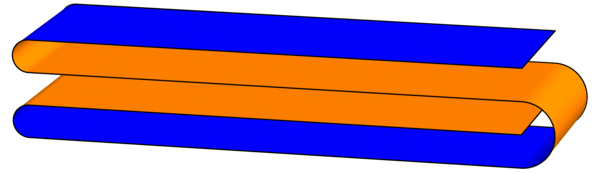As you look through the four layers from top to bottom, write down the colors you encounter, in order: the first layer shows Indigo (I), the second layer has Orange (O), and so on. The full order is IOOI, which looks suspiciously like the start of the Thue-Morse sequence. (This sequence was introduced last week.)

To continue the experiment, treat this figure as a single strip and fold the same flat S-shape again. There will be 16 layers down the middle: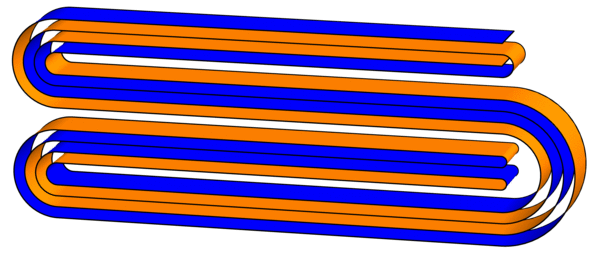Sure enough, the order of colors encountered is IOOI OIIO OIIO IOOI, which is the first 16 digits of the Thue-Morse sequence. This pattern will continue forever (or at least until the paper gets too thick to fold): every time you fold another S-shape, you quadruple the number of digits of the Thue-Morse sequence (can you see why?). Try to fold a third iteration! It looks like this (click for larger image):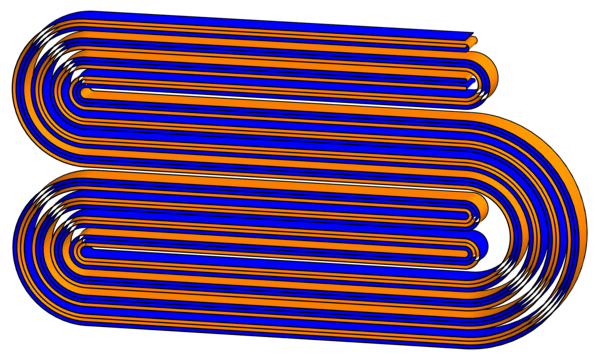But wait, there’s more! Now that we have these creased strips, what happens when we unfold them? Specifically, follow the existing creases and lay out the strips so that each crease makes a 90-degree angle. What shapes do we get? Surprisingly, they exactly fill triangular grids of squares:Can you figure out why this pattern continues?

# Thue-Morse Navigating Turtles

Here’s a simple rule to generate a pretty sequence of 1s and 0s: Start with the list 1 (just one digit) and repeatedly form a new list by flipping all the bits (1s become 0s and vice versa) and attaching this to the end: $$1 \to 10 \to 1001 \to 10010110 \to 1001011001101001 \to \cdots.$$ For example, to get from 1001 to the next sequence, we swap 1s and 0s to form 0110, and then we join these together to make 10010110. By continuing this process we can make our list longer and longer, and the limiting sequence $$1001011001101001011010011001011001101\cdots$$ is called the Thue-Morse Sequence.

What did I mean by calling this sequence “pretty”? Well, what does this sequence “look like”? One way to visualize it is with a turtle program. Imagine a turtle (let’s call him Leo) walking along the plane, and treat the digits of the Thue-Morse sequence as a list of instructions for the turtle as follows: When Leo sees the digit 1, he steps forward one foot; a 0 tells Leo to turn left by 60 degrees. What does Leo’s path look like? Here are the first 32 instructions he follows: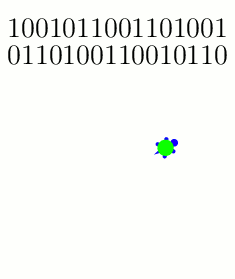The turtle follows these instructions on the first 32 entries of the Thue-Morse sequence: {1: Step; 0: Turn 60}.

And here are snapshots of his path as he gets farther into the Thue-Morse sequence: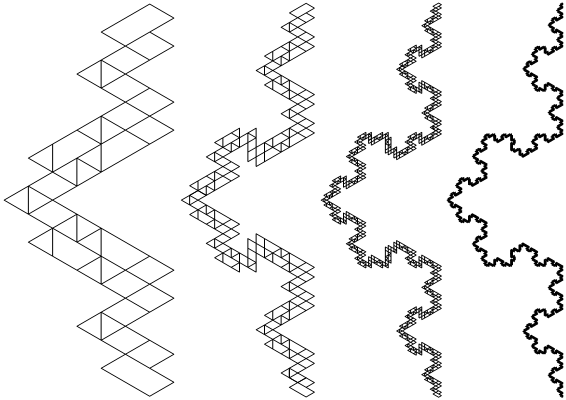Snapshots of the turtle's trajectories after 2^8, 2^10, 2^12, and 2^14 instructions, respectively.

Why did we pick these instructions? What if Leo turns 120 degrees instead of 60 degrees when he sees a 0?The turtle follows the Thue-Morse sequence with a slightly different set of instructions: {1: Step; 0: Turn 120}. Shown here after 2^6, 2^8, 2^10, and 2^12 instructions, respectively.

If you zoom out and/or squint a little, these paths are roughly following a famous fractal called the Koch curve. And indeed, the more steps you draw, the closer the resemblance will become (as analyzed in these two papers: [MH2005] and [KZH2008]). But why stop here? What happens if we change the turtle’s instructions even more? I played around with some new rule sets, and these too show how the Koch curve seems innately “programmed” into the structure of the Thue-Morse sequence:Three more pretty examples of the Thue-Morse sequence's "turtle power." In all three cases, the trajectories converge to the Koch curve. Left: {1: (Turn 180, Step); 0: (Turn 60, Step)}, after 2^8 instructions. Middle: {1: (Turn 180, Step); 0: (Turn 120, Step)}, after 2^10 instructions. Right: {1: Turn 180; 0: (Turn 15, Step)}, after 2^10 instructions.

Here’s a final challenge: if the Koch curve and the Thue-Morse sequence are so intimately related, shouldn’t we be able to find some turtle program instructions that trace the Koch curve without “squinting”? Specifically, the Koch curve is usually constructed as the limit of the following sequence of paths, where each iteration is made by stitching together four copies of the previous curve:The Koch curve is constructed as the limit of these paths, where each curve is formed from four (shrunken) copies of the previous curve.

Is there a set of turtle instructions whose path traces exactly these curves? In fact, there is! These instructions work: {1: (Step, Turn 60); 0: Turn 180}.

# Archimedes’ Circular Reasoning

Every geometry textbook has formulas for the circumference ($$C = 2 \pi r$$) and area ($$A = \pi r^2$$) of a circle. But where do these come from? How can we prove them?

Well, the first is more a definition than a theorem: the number $$\pi$$ is usually defined as the ratio of a circle’s circumference to its diameter: $$\pi = C/(2r)$$. Armed with this, we can compute the area of a circle. Archimedes’ idea (in 260 BCE) was to approximate this area by looking at regular $$n$$-sided polygons drawn inside and outside the circle, as in the diagram below. Increasing $$n$$ gives better and better approximations to the area.The n-sided regular polygons inside (blue) and outside (red) a circle can be used to approximate the area of the circle. Shown here for n=12.

Look first at the inner polygon. Its perimeter is slightly less than the circle’s circumference, $$C = 2 \pi r$$, and the height of each triangle is slightly less than $$r$$. So when reassembled as shown, the triangles form a rectangle whose area is just under $$C/2\cdot r = \pi r^2$$. Likewise, the outer polygon has area just larger than $$\pi r^2$$. As $$n$$ gets larger, these two bounds get closer and closer to $$\pi r^2$$, which is therefore the circle’s area.

Archimedes used this same idea to approximate the number $$\pi$$. Not only was he working by hand, but the notion of “square root” was not yet understood well enough to compute with. Nevertheless, he was amazingly able to use 96-sided polygons to approximate the circle! His computation included impressive dexterity with fractions: for example, instead of being able to use $$\sqrt{3}$$ directly, he had to use the (very close!) approximation $$\sqrt{3} > 265/153$$. In the end, he obtained the bounds $$3\frac{10}{71} < \pi < 3\frac{1}{7}$$, which are accurate to within 0.0013, or about .04%. (In fact, he proved the slightly stronger but uglier bounds $$3\frac{1137}{8069} < \pi < 3\frac{1335}{9347}$$. See this translation and exposition for more information on Archimedes’ methods.)

These ideas can be pushed further. Focus on a circle with radius 1. The area of the regular $$n$$-sided polygon inscribed in this circle can be used as an approximation for the circle’s area, namely $$\pi$$. This polygon has area $$A_n = n/2 \cdot \sin(360/n)$$ (prove this!). What happens when we double the number of sides? The approximation changes by a factor of $$\frac{A_{2n}}{A_n} = \frac{2\sin(180/n)}{\sin(360/n)} = \frac{1}{\cos(180/n)}.$$ Starting from $$A_4 = 2$$, we can use the above formula to compute $$A_8,A_{16},A_{32},\ldots$$, and in the limit we find that $$\pi = \frac{2}{\cos(180/4)\cdot\cos(180/8)\cdot\cos(180/16)\cdots}.$$ Finally, recalling that $$\cos(180/4) = \cos(45) = \sqrt{\frac{1}{2}}$$ and $$\cos(\theta/2) = \sqrt{\frac{1}{2}(1+\cos\theta)}$$ (whenever $$\cos(\theta/2) \ge 0$$), we can rearrange this into the fun infinite product $$\frac{2}{\pi} = \sqrt{\frac{1}{2}} \cdot \sqrt{\frac{1}{2}+\frac{1}{2}\sqrt{\frac{1}{2}}} \cdot \sqrt{\frac{1}{2}+\frac{1}{2}\sqrt{\frac{1}{2}+\frac{1}{2}\sqrt{\frac{1}{2}}}} \cdots$$ (which I found at Mathworld). (It’s ironic that this formula for a circle uses so many square roots!)

# Astounding “Natural” Identities

The modest sequence $$1,2,3,4,\ldots$$ can do some rather awe-inspiring things, when properly arranged. Here’s a short list of some of its many impressive feats.

There are numerous expressions for $$\pi$$ relying on the progression of integers, including the Wallis formula: $$\frac{\pi}{2} = \frac{2}{1} \cdot \frac{2}{3} \cdot \frac{4}{3} \cdot \frac{4}{5} \cdot \frac{6}{5} \cdot \frac{6}{7} \cdots$$ (which can be derived from Complex Analysis using an infinite product representation for the sine function) and an elegant alternating sum: $$\frac{\pi-3}{4} = \frac{1}{2\cdot 3\cdot 4}-\frac{1}{4\cdot 5\cdot 6}+\frac{1}{6\cdot 7\cdot 8}-\cdots$$ (try to prove this!). Euler’s number $$e$$ has similarly surprising formulas, such as $$\frac{1}{e-2} = 1+\frac{1}{2+\frac{2}{3+\frac{3}{4+\frac{4}{5+\cdots}}}}$$ (listed at MathWorld) and $$\frac{e}{e-1} = 1+\frac{1+\frac{1+\frac{1+\cdots}{2+\cdots}}{2+\frac{2+\cdots}{3+\cdots}}}{2+\frac{2+\frac{2+\cdots}{3+\cdots}}{3+\frac{3+\cdots}{4+\cdots}}}$$ (which is problem 1745 in Mathematics Magazine, posed by Gerald A. Edgar).

The list doesn’t stop here! The nested square-root identity $$3 = \sqrt{1+2\sqrt{1+3\sqrt{1+4\sqrt{1+\cdots}}}}$$ is attributed to Ramanujan (on this Wikipedia page). As another curiosity, the sequence $$\frac{1}{2},\qquad \frac{1}{2} \Big/ \frac{3}{4},\qquad \frac{\frac{1}{2} \big/ \frac{3}{4}}{\frac{5}{6} \big/ \frac{7}{8}},\qquad \frac{\frac{1}{2} \big/ \frac{3}{4}}{\frac{5}{6} \big/ \frac{7}{8}} \bigg/ \frac{\frac{9}{10} \big/ \frac{11}{12}}{\frac{13}{14} \big/ \frac{15}{16}},\qquad\ldots$$ (which relates to the Thue-Morse sequence) can be shown to converge to $$\sqrt{2}/2$$.

There are many pretty/unexpected/crazy formulas obtainable from the natural numbers $$1,2,3,4,\ldots$$ that could not fit in this post. What are some of your favorites?

# Some Strange Sums

If someone told you to compute the value of the infinite sum $$Z = 1+2+3+4+\cdots$$, you would tell them that it simply does not converge. But what if they insisted that you assign a finite value to this sum? Which value would you give? Is there any way to make sense of this question? Yes, and the answer is $$-1/12$$.

Before tackling this sum, let’s try a slightly easier one, known as Grandi’s Series: $$G = 1-1+1-1+\cdots$$. How can we compute its value? Let’s write \begin{align*}G &= 1-1+1-1+1-\cdots \text{ and}\\ G &= 0+1-1+1-1+\ldots,\end{align*} and adding these term by term gives $$2G = 1+0+0+0+0+\cdots = 1$$, i.e., $$G = 1/2$$. So we got a value! The same “trick” lets us sum $$H = 1-2+3-4+\cdots$$ like this: \begin{align*}2H &= 1 + (-2+1) + (3-2) + (-4+3) + \cdots\\ &= 1-1+1-1+\cdots\\ &= G = 1/2,\end{align*} so $$H = 1/4$$.

What’s really going on here? A good way to understand it is to look at the functions $$g(x) = 1-x+x^2-x^3+\cdots$$ and $$h(x) = 1-2x+3x^2-4x^3+\cdots$$, and to realize that we’re asking for $$g(1)$$ and $$h(1)$$, respectively. The sum defining $$g(x)$$ converges for $$-1 < x < 1[/latex], and since $g(x) + x \cdot g(x) = 1$ (this is just the "trick" from above!), we find $g(x) = 1/(1+x)$. So, even though the sum defining $g(1) = 1-1+1-\cdots$ does not converge, the limit as $x$ approaches 1 from below is well defined and equals $1/(1+1) = 1/2$. Likewise, we find that $h(x) = 1/(1+x)^2$ for $-1 < x < 1$, and the limit as $x$ goes to 1 is 1/4. This method of putting the terms as the coefficients of a power series and extrapolating with limits is called Abel Regularization. Notice, however, that this method does not work for the sum [latex]Z$$, because $$1 + 2x + 3x^2 + 4x^3 + \cdots = 1/(1-x)^2$$, and the limit as $$x$$ approaches 1 is still infinite.

A different method of summing divergent series is called Zeta Function Regularization: to compute $$a_1 + a_2 + a_3 + \cdots$$, define the function $$a_1^{-s} + a_2^{-s} + a_3^{-s} + \cdots$$ (which hopefully converges for large enough s), and try to “extend” this to a function we can evaluate at $$s=-1$$. For example, for $$H = 1-2+3-\cdots$$, the corresponding function $$1/1^2-1/2^s+1/3^s-\cdots$$ is called the Dirichlet Eta Function, $$\eta(s)$$. Analysis based on our “trick” from above (iterated infinitely many times! [producing the so-called Euler Transform]) can be used to show that $$\eta(s)$$ can be defined for all values $$s$$ (even complex values!) and that $$\eta(-1) = 1/4$$, which agrees with our computation of $$1-2+3-4+\cdots$$ above. The corresponding function for the sum $$Z$$ is called the Riemann Zeta Function, $$\zeta(s)$$, and we can compute (for all $$s$$ where this makes sense): $$\begin{gather*} \zeta(s) = \frac{1}{1^s} + \frac{1}{2^s} + \frac{1}{3^s} + \frac{1}{4^s} + \cdots,\\ 2^{-s} \zeta(s) = \frac{1}{2^s} + \frac{1}{4^s} + \frac{1}{6^s} + \frac{1}{8^s} + \cdots, \text{ and so}\\ \zeta(s)-2\cdot 2^{-s} \zeta(s) = \frac{1}{1^s}-\frac{1}{2^s}+\frac{1}{3^s}-\frac{1}{4^s}+\cdots = \eta(s).\end{gather*}$$ So $$(1-2^{1-s}) \zeta(s)$$ equals $$\eta(s)$$ everywhere (by general complex analysis facts). At $$s = -1$$, this says $$\zeta(-1) = \eta(-1)/(-3) = -1/12$$, as claimed.

# A “Frac”ing Prime Generator

Last week we saw a few ways to (try to) generate prime numbers with simple formulas. I will now describe a much more puzzling (and recreational) method. The following numbers specify everything you need: $$\left(\frac{17}{91}, \frac{78}{85}, \frac{19}{51}, \frac{23}{38}, \frac{29}{33}, \frac{77}{29}, \frac{95}{23}, \frac{77}{19}, \frac{1}{17}, \frac{11}{13}, \frac{13}{11}, \frac{15}{14}, \frac{15}{2}, \frac{55}{1} \right).$$ To run this “game,” the rules are as follows: Start with value $$v = 2$$. At each step, find the first fraction $$f$$ in the list so that $$v \cdot f$$ is an integer, and replace the value $$v$$ by this integer. Repeat indefinitely (or until no such $$f$$ can be found). The beginning of the computation looks like this:

1. Start with value $$v = 2$$.
2. The first fraction $$f$$ in the list such that $$2\cdot f$$ is an integer is $$f=15/2$$, so the new value $$v$$ is $$2 \cdot 15/2 = 15$$.
3. The first $$f$$ in the list with $$15 \cdot f$$ an integer is $$f=55/1$$, so the new $$v$$ is $$15 \cdot 55/1 = 825$$.
4. The first $$f$$ with $$825 \cdot f$$ an integer is $$29/33$$, so set $$v = 825 \cdot 29/33 = 725$$.

And so on.

Continuing as above, the list of values looks like 2, 15, 825, 725, 1925, 2275, 425, 390, 330, 290, 770, 910, 170, 156, 132, 116, 308, 364, 68, 4, 30, 225, 12375, … .

But what does this have to do with primes? The list of fractions was carefully constructed so that many powers of 2 will appear, in exactly the following order: $$2^1, 2^2, 2^3, 2^5, 2^7, 2^{11}, 2^{13}, 2^{17}, \ldots .$$ Indeed, except for $$2^1$$ (the starting value), any power of two obtained by this process will have a prime exponent, and all primes will appear in this way (in order)! This is a very special property of the chosen list of fractions, and different lists give rise to vastly different output sequences.

In fact, you can think of this procedure as a programming language, where the list of fractions and the starting value constitute your “program.” This language is called FRACTRAN, and both the language and the above prime-enumerating program were designed by John Conway. What else is this language capable of computing? Lots! FRACTRAN can compute anything that modern computers could—but much less efficiently. (In Theoretical Computer Science terminology, FRACTRAN is turing complete.) See the Wikipedia page for more information, as well as an explanation of why the above program works.